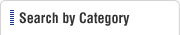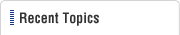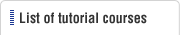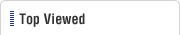HOME  > Low Cost Automation Tutorial  > #031 Motion Mechanism Design - 1 : Ballscrew Selection
Low Cost Automation Tutorial

#031 Motion Mechanism Design - 1 : Ballscrew Selection

Category : Drive mechanism design
February26, 2010

Major contributing factors for selecting ballscrews are:1 Mass of the work and the table (m) (moving objects)2 Feed speed3 Time constant

The 2. and 3. above determine the acceleration (α). Therefore, it can be understood that the moving mass (m) and acceleration (α) are related to selecting ballscrews. This means that it is important to select proper ballscrews in relation to the linear force (F) generated according to Newton's Second Law: F=mα Furthermore, inertial moments (equivalent to mass (m) of rotary motion, see tutorial #111) will affect the driven object during an interval of staring and stopping motions.

Maximum reduction of driven mass (m) and acceleration (α), which will give the most flexibility in ballscrew selection, is where the LCA designer's abilities are measured.

Explanation on technical terms for ballscrew selection

Explanation on technical terms for ballscrew selection

Allowable rotational speed: DN Value

Determined by a product of multiplying ball diameter D (mm) and shaft rotational speed N (rpm). Typical DN value ≦50000〜70000.

Critical speed

When the shaft's angular velocity corresponds with its resonant frequency, an oscillation begins and the shaft will rotate with increased wobble. This causes the shaft to distort. The speed which this occurs is called the Critical Speed.

(Feed screw's) Rigidity

The rigidity can be expressed and understood as same as spring constant. When the whole mechanism is considered as a spring system, the load (P) and the deformation (Λ) are in a proportional relationship, and the inverse of this proportional constant is called the Rigidity. When the mechanism is defined as a spring, this is called Hook's Law and its proportional constant is an elastic coefficient.

Time constant

This is a value indicating the time it takes for the system to reach a targeted control condition (defined as the motor's slew speed in MISUMI catalog), in other words the Rise Time. Small time constant will mean a control that can reach the targeted speed in a short time, which in the control terms requires a higher acceleration.